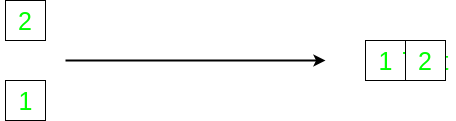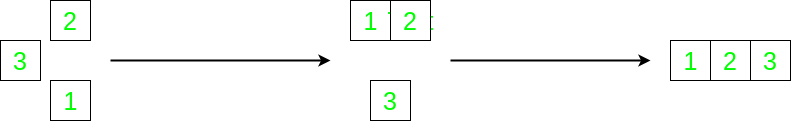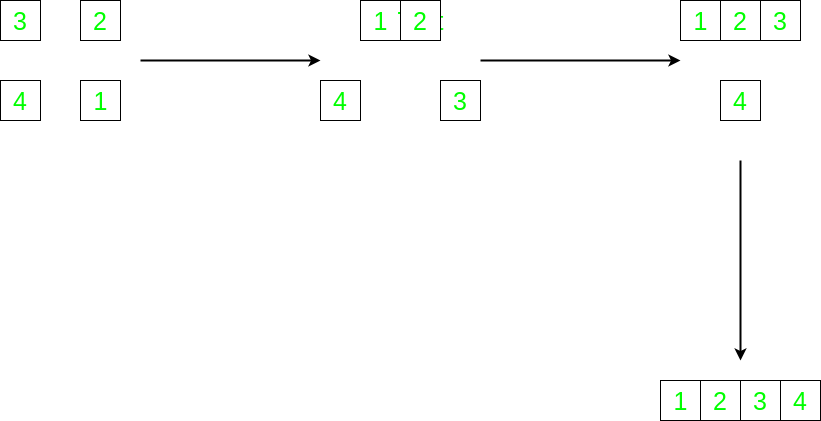Related Articles
Minimum number of moves required to solve a Jigsaw Assembly Puzzle
• Difficulty Level : Basic
• Last Updated : 31 Mar, 2020

Puzzle:
You are given a jigsaw puzzle that contains n pieces. A “section” of the puzzle is a set of one or more pieces that have been connected to each other. A “move” consists of connecting two sections. Given that initially, all the pieces are separate, then what is the minimum number of moves in which the puzzle can be completed?

Answer

Let’s consider the above problem case-wise:

1. Case n = 2: In this case, we only have to combine two pieces, which can be done in a single move. Hence, the total number of moves required is 1.2. Case n = 3: Here, we can first join any two pieces as required and then, we can add the final piece to the section. This results in the total number of 2 moves.3. Case n = 4: Just continuing from the last case, we can first combine two pieces, then combine the third one and finally, the fourth piece. The total number of moves required in this case is 3.4. For any value of n: Notice, the pattern building up in the previous 3 cases. We first solve the puzzle for (n-1) pieces and then finally add the last piece with 1 move.

Let’s represent this mathematically:Hence,

The minimum number of moves required to solve n-piece jigsaw puzzle is (n-1)

Attention reader! Don’t stop learning now. Get hold of all the important DSA concepts with the DSA Self Paced Course at a student-friendly price and become industry ready.

My Personal Notes arrow_drop_up